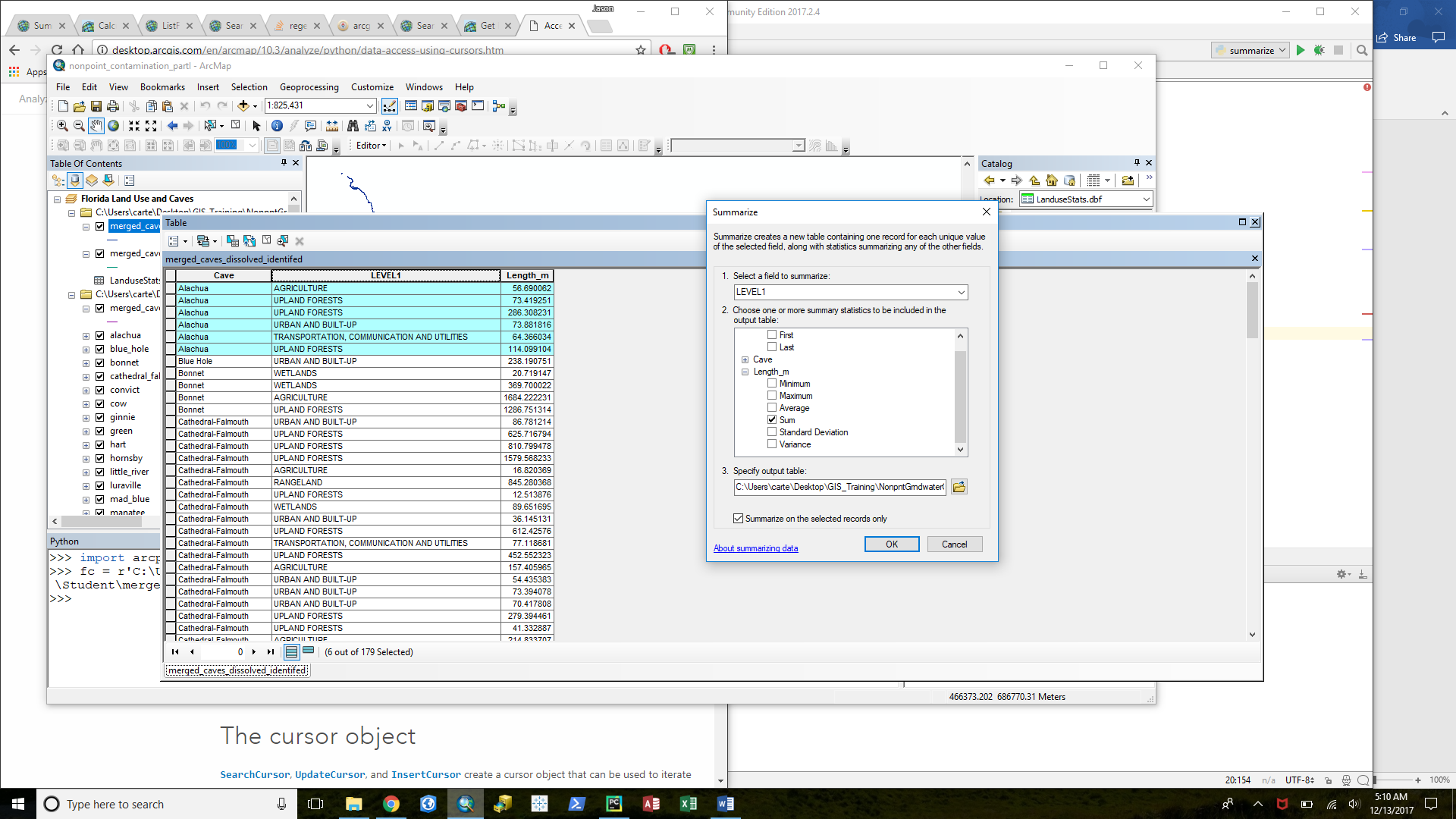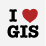# How to summarize field statistics in arcpy using a SearchCursor?

2392
2
12-12-2017 09:25 PMNew Contributor III

Hi,

I'm working on tutorial data that has a table structured as shown in the photo below. The tutorial is asking that I summarize the length of each Cave (selected feature) in each Landuse manually using the Summarize tool from the Attributes Table and create a separate table.dbf for each Cave. I'm trying to do this in Python and I'm running into an error. Also, I could be taking the wrong approach. Any advice much appreciated. Thank you!

Here is the table:Here is my code:

``````import arcpy
import os

fc = r'C:\Users\xxxx\Desktop\GIS_Training\NonpntGrndwaterContCaves_2013\NonpntGrndwaterContCaves_2013\Student\merged_caves_dissolved_identifed.shp'
fld_luse = 'LEVEL1'
fld_length = 'Length_m'
fld_cave = 'Cave'

caves = list(set(r for r in arcpy.da.SearchCursor(fc,fld_cave)))

summaryFields = ['fld_luse']
summaryStats = [fld_length, 'SUM']
fc2 = r"C:\Users\xxx\Desktop\GIS_Training\NonpntGrndwaterContCaves_2013\NonpntGrndwaterContCaves_2013\Student\\"

for cave in caves:
where = '"{0}" = \'{1}\''.format(fld_cave, cave)
caveObj = arcpy.da.SearchCursor(fc, where, "Cave; LEVEL1; Length_m")
outFS = os.path.join(os.path.dirname(fc2), cave + '.dbf')
arcpy.Statistics_analysis(in_table=caveObj, out_table=outFS, statistics_fields="{0} SUM;{0} MEAN;{0} MIN;{0} MAX;{0} RANGE;{0} COUNT;{0} STD".format(fld_length))
‍‍‍‍‍‍‍‍‍‍‍‍‍‍‍‍‍‍‍‍‍‍‍‍‍‍‍‍‍‍‍‍‍‍‍‍‍‍‍``````

And the error I am receiving:

``````Traceback (most recent call last):
File "C:/Users/xxx/Desktop/GIS_Training/NonpntGrndwaterContCaves_2013/NonpntGrndwaterContCaves_2013/Student/summarize.py", line 20, in <module>
arcpy.Statistics_analysis(in_table=caveObj, out_table=outFS, statistics_fields="{0} SUM;{0} MEAN;{0} MIN;{0} MAX;{0} RANGE;{0} COUNT;{0} STD".format(fld_length))
File "C:\Program Files\ArcGIS\Pro\Resources\ArcPy\arcpy\analysis.py", line 1695, in Statistics
raise e
File "C:\Program Files\ArcGIS\Pro\Resources\ArcPy\arcpy\analysis.py", line 1692, in Statistics
retval = convertArcObjectToPythonObject(gp.Statistics_analysis(*gp_fixargs((in_table, out_table, statistics_fields, case_field), True)))
File "C:\Program Files\ArcGIS\Pro\Resources\ArcPy\arcpy\geoprocessing\_base.py", line 506, in <lambda>
return lambda *args: val(*gp_fixargs(args, True))
RuntimeError: Object: Error in executing tool‍‍‍‍‍‍‍‍‍‍‍‍‍‍‍‍‍‍‍‍``````
1 Solution

Accepted SolutionsbyEsri Regular Contributor

Hi

I do not think you can give search cursor as a parameter to Statistics

You can try to MakeFeatureLayer with the where and use it in statistics.

2 RepliesbyEsri Regular Contributor

Hi

I do not think you can give search cursor as a parameter to Statistics

You can try to MakeFeatureLayer with the where and use it in statistics.New Contributor III

Awesome, thanks!